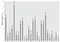Our secret messages: Caesar Cypher

Let´s talk about encryption, that useful thing that we know that a lot of devices work with but seems complicated. A lot of centuries ago the humanity note the necessity of transmit a message but the only person who should know the content is the receiver.

Introduction

A genius but terrible man create one of the first encryption methods, the Caesar method. Julius Caesar send his messages with shifts in the alphabet. This cypher have the characteristic that is symmetric, this mean that the receiver and transmitter needs the password or key know the secret. The amount of shift letters is apply to every letter in the message and this amount is the key in this cypher. For being more clear, an example with a code in python:

Application

Our message will be “alphabets” and the amount of shifts will be 12. With this conditions the letters “A” will be the letter “M” and so on. The encrypted message is “mxbtmnqfe”.

The concept is simple but is more interesting to put this cypher in the language of the nature, mathematics. To begin this road, we convert the letters into numbers and for this it is necessary to use a reference, in this case the English alphabet with 26 characters. For the sake of the code the initial number is 0 not 1.

After convert the numbers, then sum the shift, in this case 12 with every letter in the message.

Notice that are numbers larger than the maximum length of the alphabet, so we appeal to a mathematical operation call module. The characteristic of this operation is the circular shift, this mean that when we reach the maximum length plus one, it restart the count, so 26 it is 0. Then the message follow like this:

Finally we traduce the numbers to letters.

Code

The next code illustrates the cypher:

Breaking the cypher

Among the ways to break a cipher there are two that are very interesting in this occasion. The analytical way and the brute force attack. For the analytical way, there is a graphic that illustrate the probability of the letters in the English vocabulary. With this data is possible to decipher a message take into account the association between letter by probability.Probability of each letter: Relative frequency (%) vs Letters. Taken from Cryptography and Network Security — William Stallings

If there is enough cipher text to analyze then is possible to deduce the key or the amount of shift letters. It can be done by counting the most common letter in average and suppose that is an “e” , then the second most common letter in average and suppose that is a “t”, and so on. After this process the message can be decipher.

The other way is the brute force way and consist in testing all the possible keys within the cipher message, this method is used when is possible to probe many combination with high speed and a processor can do this very easy.

| PUJ Electronic Enginer | Python programmer |